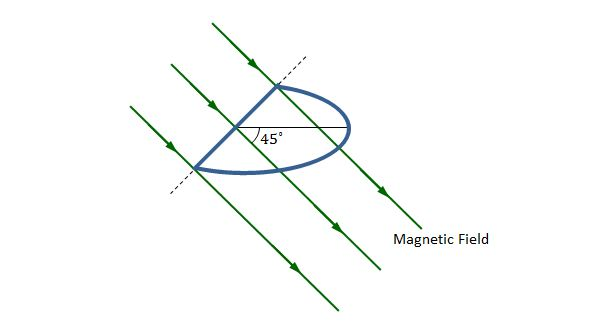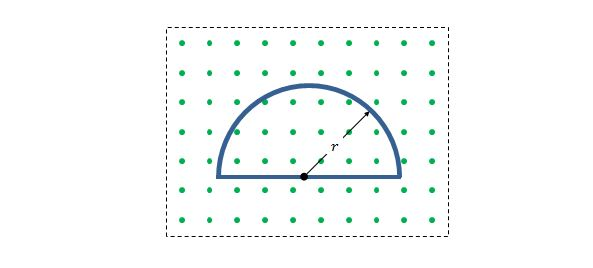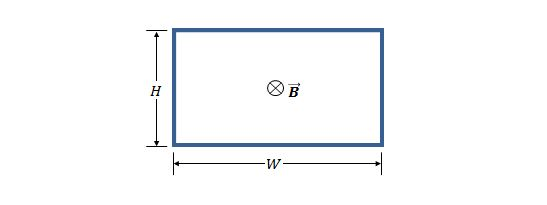Electricity and Magnetism

# Magnetic flux and Faraday's law (quantitative)A closed loop of wire that consists of a semicircle of radius $3.2\text{ cm}$ and a straight line, as shown in the above figure, is lying in a uniform magnetic field $\vec{B}$ of magnitude $74\text{ mT}.$ The magnetic field is perpendicular to the straight line of the loop and makes an angle of $45^\circ$ with the plane of the semicircle. If the magnetic field is reduced to zero at a uniform rate during a time interval of $6.0\text{ ms},$ what is the approximate magnitude of the electromotive force induced in the loop during this interval?

Suppose that an elastic conducting material, which is placed in a uniform $0.800\text{ T}$ magnetic field, is stretched into a circle loop with radius $15.0\text{ cm}.$ The plane of the loop is perpendicular to the magnetic field, and the stretched loop is released to shrink at an instantaneous rate of $75.0\text{ cm/s}.$ What is the approximate magnitude of the electromotive force induced in the shrinking loop?

A circular wire loop with radius $0.30\text{ m}$ is lying in a magnetic field with magnitude $0.50\text{ T}.$ The plane of the loop is perpendicular to the magnetic field. If the direction of the magnetic field is reversed and its magnitude is reduced to $0.20\text{ T}$ in $2.0\text{ s},$ what is the approximate magnitude of the average induced electromotive force in the loop during this time interval?Consider a conducting loop of a half-circle of radius $r=0.30\text{ m},$ as shown in the above figure. The loop lies in a uniform magnetic field $\vec{B}$ that is directed out of the screen. If the field magnitude is given by $B=4.0t^2+4.0t+3.0,$ where $B$ is in teslas and $t$ is in seconds, what is the approximate magnitude of the electromotive force induced around the loop by that field $\vec{B}$ at $t=5\text{ s}?$A rectangular loop of wire is immersed in a nonuniform and varying magnetic field $\vec{B}$ that is perpendicular to and directed into the screen, as shown in the above figure. The loop has width $W=3.0\text{ m}$ and height $H=2.0\text{ m}.$ If the magnitude of the magnetic field is given by $B=5t^2x^2,$ where $B$ is in teslas, $t$ is in seconds, and $x$ is in meters, what is the magnitude of the induced electromotive force around the loop at $t=0.30\text{ s}?$

×